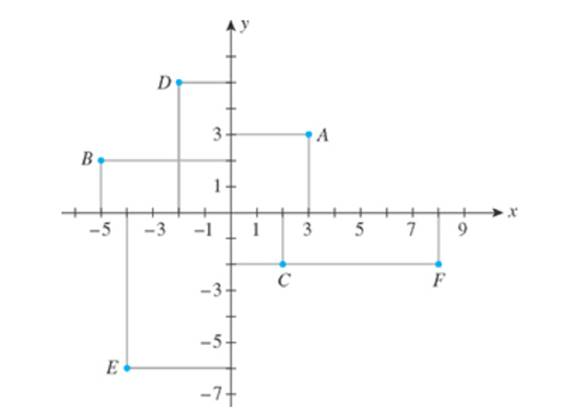Chapter 1.3, Problem 6E

Chapter
Section
Textbook Problem

In Exercises 1-6, refer to the following figure, and determine the coordinates of each point and the quadrant in which it is located.6. F

To determine
The coordinate of point F and the quadrant in which it is located.

Explanation

Given:

The figure is,

Figure (1)

Calculation:

From the given Figure (1), the point F cuts x-axis vertically at 8 and cuts y-axis horizontally at 2

Still sussing out bartleby?

Check out a sample textbook solution.

See a sample solution

The Solution to Your Study Problems

Bartleby provides explanations to thousands of textbook problems written by our experts, many with advanced degrees!

Get Started

Find more solutions based on key concepts